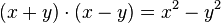# Mental calculation facts for kids

Kids Encyclopedia Facts

Mental calculation or mental maths is an old mathematical tool. It is doing maths without using any tools or writing anything down. Mental calculation uses only the human brain. People who use mental maths use shortcuts to make the calculation easier. People who do mental calculation do it without a computer or a calculator.

Mental calculation often involves the use of specific techniques devised for specific types of problems. There are specific methods for adding, subtracting, multiplying or dividing and more calculations such as squaring numbers or its opposite [square] "root finding". And even specific methods depending on the numbers used, such as "multiply by 10" or "multiply any 2-digit numbers", or "square numbers near 50".

## Calculation methods

There are many methods to do mental operations such as multiplying.

To multiply an integer by 10, simply add an extra 0 to the end of the number. To multiply a non-integer by 10, move the decimal point to the right one digit. In general

Another way to do multiplications with two numbers is to use binomial formulas.$(x+y) \cdot (x-y)= x^2 - y^2$Mental calculation Facts for Kids. Kiddle Encyclopedia.